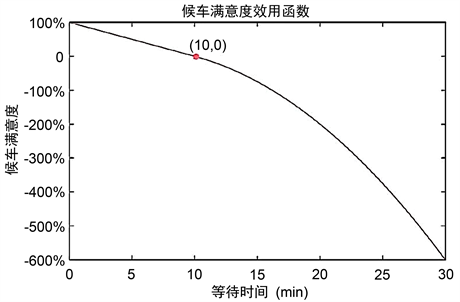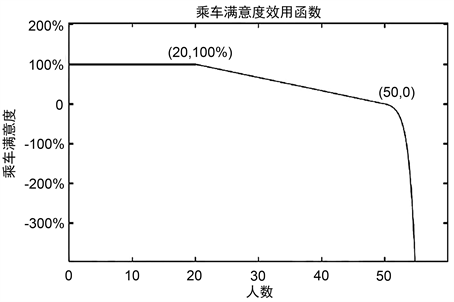# 曲阜市电动公交车的运行路线及换车策略建模分析Modeling and Analysis of Operation Route and Change Strategy of Electric Bus in Qufu

DOI: 10.12677/ORF.2020.103020, PDF, HTML, XML, 下载: 26  浏览: 62  国家科技经费支持

Abstract: With the increasing attention of the society to the problem of urban pollution, electric buses are widely used because of their low noise and zero emission. To formulate a reasonable scheduling scheme for electric buses, the paper based on historical data, from the interests of passengers and bus companies, with the average full load rate, passenger comfort and satisfaction as the goal, the optimization model of the scheduling scheme is set up to give the ideal departure schedule and the minimum number of vehicles by solving the model.

1. 引言

2. 公交车调度模型

1) 两辆公交车发车相差时间要取整数，并且在行驶过程中，相互追赶不上。

2) 假设在各个时间段内各站点的等待上车的人数服从泊松分布且数据真实可靠。

3) 交通良好，无堵车现象，路面平坦，无车辆损毁现象以及无其它意外情况 。

4) 公交车在行驶过程中做匀速运动，公交车的发车时间固定，严格按照时刻表，发车数量也固定 。

C：每日所需总车次数； ${C}_{ij}$ ：上行或下行第j时段的车次；

${S}_{ij}$ ：上行或下行第j时段平均发车时差； ${P}_{ij}$ ：上行或下行第j时段平均载客量；

${T}_{ij}$ ：上行或下行的平均发车时间间隔； ${M}_{gi}$ ：上行或下行时公交公司日平均满意度；

${M}_{ci}$ ：上行或下行时乘客整体日平均满意度； ${M}_{gij}$ ：上行或下行时公交公司各时段的满意度；

${M}_{cij}$ ：上行或下行时乘客各时段的满意度；Q：每日所需车辆数；

B：满载率上限；b：乘客候车的时间；

${d}_{m}^{+}$ ：为正偏差变量； ${d}_{m}^{-}$ ：负偏差变量； ${w}_{k}$ ：权重。

$i=2$ (表示下行运动， $k=1,2,3,\cdots ,13$$j=1,2,3,\cdots ,12$ )。

2.1. 最大客运量模型Table 1. Up and down each moment of maximum passenger capacity

2.2. 公交车调度最小车辆数问题

$\mathrm{min}Z={w}_{1}{d}_{1}+{w}_{2}{d}_{2}+{w}_{3}{d}_{3}+{w}_{4}{d}_{4}$

$\left\{\begin{array}{l}x+{d}_{1}^{-}-{d}_{1}^{+}=b\\ x+{d}_{2}^{-}-{d}_{2}^{+}=1\\ {f}_{i}\left(x\right)+{d}_{3}^{-}-{d}_{3}^{+}=120,\left(i=1,2,\cdots ,13\right)\\ {f}_{j}\left(x\right)+{d}_{3}^{-}-{d}_{3}^{+}=50,\left(j=1,2,\cdots ,13\right)\\ {d}_{m}^{-},{d}_{m}^{+}\ge 0,\left(m=1,2,3,4\right)\end{array}$

2.3. 乘客满意度模型

2.3.1. 刻画乘客满意度的依据

2.3.2. 乘客等待时间的影响

${W}_{i,j}\left(h\right),h=1,2,\cdots ,{h}_{i,j}$

$T{W}_{i,j}\left(h\right)={T}_{i,j}-{T}_{i-h,j},h=1,2,\cdots ,{h}_{i,j}$Figure 1. The utility function of passenger waiting satisfaction

${P}_{1}\left(T\right)=\underset{{T}_{i,j}\in \Lambda }{\sum }\left\{{\mu }_{1}\left({W}_{i,j}\left(h\right)\right)\right\}=\underset{{T}_{i,j}\in \Lambda }{\sum }\left\{{\mu }_{1}\left({T}_{i,j}-{T}_{i-h,j}\right)\right\}$

${P}_{2}\left(T\right)=\underset{{T}_{i,j}\notin \Lambda }{\sum }\left\{{\mu }_{1}\left({W}_{i,j}\left(h\right)\right)\right\}=\underset{{T}_{i,j}\notin \Lambda }{\sum }\left\{{\mu }_{1}\left({T}_{i,j}-{T}_{i-h,j}\right)\right\}$

${\mu }_{1}\left(x\right)=\left\{\begin{array}{l}1-\frac{x}{{T}^{m}},\text{\hspace{0.17em}}\text{\hspace{0.17em}}\text{\hspace{0.17em}}\text{\hspace{0.17em}}\text{\hspace{0.17em}}\text{\hspace{0.17em}}\text{\hspace{0.17em}}\text{\hspace{0.17em}}\text{\hspace{0.17em}}\text{\hspace{0.17em}}0\le x\le {T}^{m},\\ -{a}_{1}{\left(x-{T}^{m}\right)}^{2},\text{\hspace{0.17em}}\text{\hspace{0.17em}}\text{\hspace{0.17em}}x>{T}^{m},\end{array}$

${\mu }_{2}\left(x\right)=\left\{\begin{array}{l}1-\frac{x}{{T}^{mm}},\text{\hspace{0.17em}}\text{\hspace{0.17em}}\text{\hspace{0.17em}}\text{\hspace{0.17em}}\text{\hspace{0.17em}}\text{\hspace{0.17em}}\text{\hspace{0.17em}}\text{\hspace{0.17em}}\text{\hspace{0.17em}}\text{\hspace{0.17em}}\text{\hspace{0.17em}}\text{\hspace{0.17em}}\text{\hspace{0.17em}}0\le x\le {T}^{mm},\\ -{a}_{2}{\left(x-{T}^{mm}\right)}^{2},\text{\hspace{0.17em}}\text{\hspace{0.17em}}\text{\hspace{0.17em}}\text{\hspace{0.17em}}\text{\hspace{0.17em}}\text{\hspace{0.17em}}x>{T}^{mm}.\end{array}$

$0\le x\le {T}^{m}\left({T}^{mm}\right)$ 时， ${\mu }_{1}\left(x\right)\left({\mu }_{2}\left(x\right)\right)$ 线性递减；当 $x={T}^{m}\left({T}^{mm}\right)$ 时， ${\mu }_{1}\left(x\right)=0\left({\mu }_{2}\left(x\right)=0\right)$ ；当 $x>{T}^{m}\left({T}^{mm}\right)$ 时， ${\mu }_{1}\left(x\right)\left({\mu }_{2}\left(x\right)\right)$ 二次递减。当然这里 ${a}_{1}>{a}_{2}$，也就是说当乘客的等车时间超过了心理的预期之后，随着等待时间的越来越长，高峰时段相比于非高峰时段，乘客满意度下滑速度更迅速。

2.3.3. 刻画拥挤度的影响

${\mu }_{2}\left(x\right)=\left\{\begin{array}{l}1,\text{\hspace{0.17em}}\text{\hspace{0.17em}}\text{\hspace{0.17em}}\text{\hspace{0.17em}}\text{\hspace{0.17em}}\text{\hspace{0.17em}}\text{\hspace{0.17em}}\text{\hspace{0.17em}}\text{\hspace{0.17em}}\text{\hspace{0.17em}}\text{\hspace{0.17em}}\text{\hspace{0.17em}}\text{\hspace{0.17em}}\text{\hspace{0.17em}}\text{\hspace{0.17em}}\text{\hspace{0.17em}}\text{\hspace{0.17em}}x\le M,\\ -ax+c,\text{\hspace{0.17em}}\text{\hspace{0.17em}}\text{\hspace{0.17em}}\text{\hspace{0.17em}}\text{\hspace{0.17em}}\text{\hspace{0.17em}}Mb.\end{array}$Figure 2. The utility function of ride satisfaction

$Q\left(T\right)=\underset{i,j}{\sum }\left\{{\mu }_{3}\left({X}_{i,j}\right)\right\}$

2.3.4. 乘客满意度综合模型的建立

${\sum }_{{T}_{i,j}\in \Lambda }\left\{{\mu }_{1}\left({W}_{i,j}\left(h\right)\right)\right\}$${\sum }_{{T}_{i,j}\notin \Lambda }\left\{{\mu }_{1}\left({W}_{i,j}\left(h\right)\right)\right\}$${\sum }_{i,j}\left\{{\mu }_{3}\left({X}_{i,j}\right)\right\}$

$\mathrm{max}{k}_{1}\underset{{T}_{i,j}\in \Lambda }{\sum }\left\{{\mu }_{1}\left({W}_{i,j}\left(h\right)\right)\right\}+{k}_{2}\underset{{T}_{i,j}\notin \Lambda }{\sum }\left\{{\mu }_{1}\left({W}_{i,j}\left(h\right)\right)\right\}+{k}_{3}\underset{i,j}{\sum }\left\{{\mu }_{3}\left({X}_{i,j}\right)\right\}$

3. 优化模型进行求解的算法

4. 总结

NOTES

*通讯作者。

  张韵华. Mathematica符号计算系统实用教程[M]. 合肥: 科技大学出版社, 1998.  武斌. 基于遗传算法的公交调度模糊最优解[J]. 中国石油大学胜利学院学报, 2019(2): 50-53.  陈岳川, 周静, 蒋明铭, 赵宇, 杨振国. 电动公交车电池续航预测研究[J]. 城市公共交通, 2017(8): 22-26.  陈丽娟, 秦萌, 顾少平, 钱科军, 许晓慧. 计及电池损耗的电动公交车参与V2G的优化调度策略[J]. 电力系统自动化, 2020(4): 52-62.  陈识为. 纯电动公交车电传动系统研究[D]: [硕士学位论文]. 成都: 西南交通大学, 2012.  衣红明. 电动公交车电源管理系统的研究[D]: [硕士学位论文]. 济南: 齐鲁工业大学, 2013.  杨扬, 关伟, 马继辉. 基于列生成算法的电动公交车辆调度计划优化研究[J]. 交通运输系统工程与信息, 2016, 16(5): 198-204.  刘超, 谢建新, 蔡东岭. 青岛公交车智能调度系统的优化研究[J]. 汽车实用技术, 2019(10): 215-217.  吴建国. 数学建模案例精编[M]. 北京: 中国水利水电出版社, 2005.  白其峥. 数学建模案例分析[M]. 北京: 海洋出版社, 2000.  赵静, 但琦. 数学建模与数学实验[M]. 北京: 高等教育出版社, 2014.  姜启源. 数学模型[M]. 北京: 高等教育出版社, 1986.  杨启帆. 数学建模[M]. 北京: 高等教育出版社, 2005.